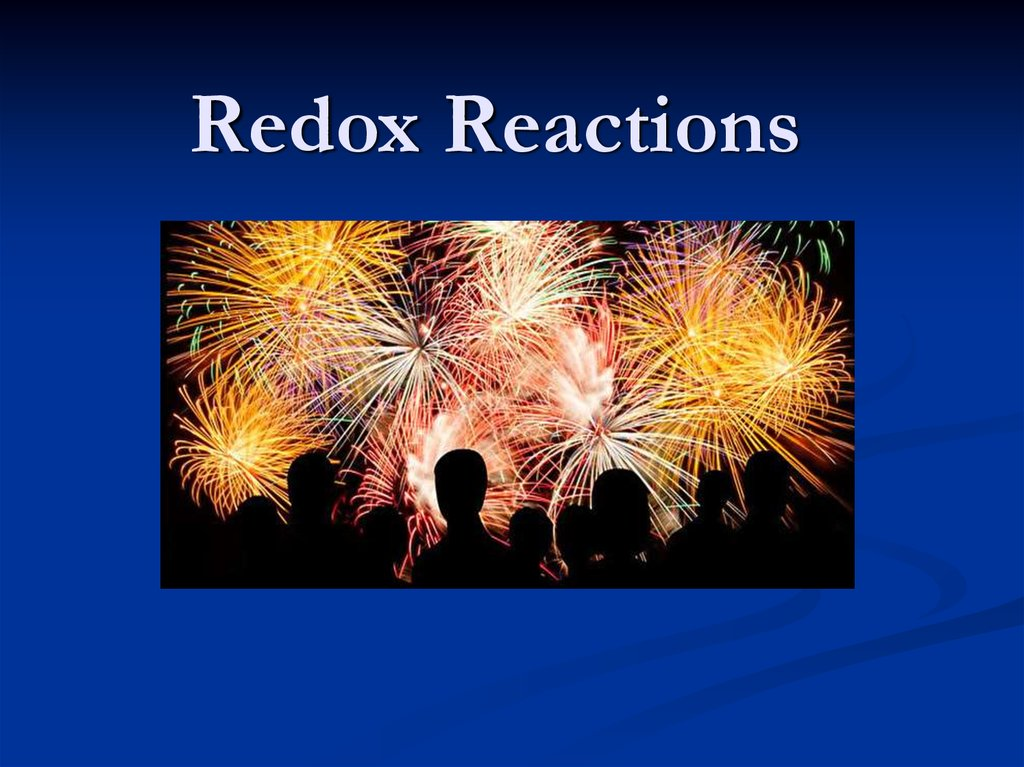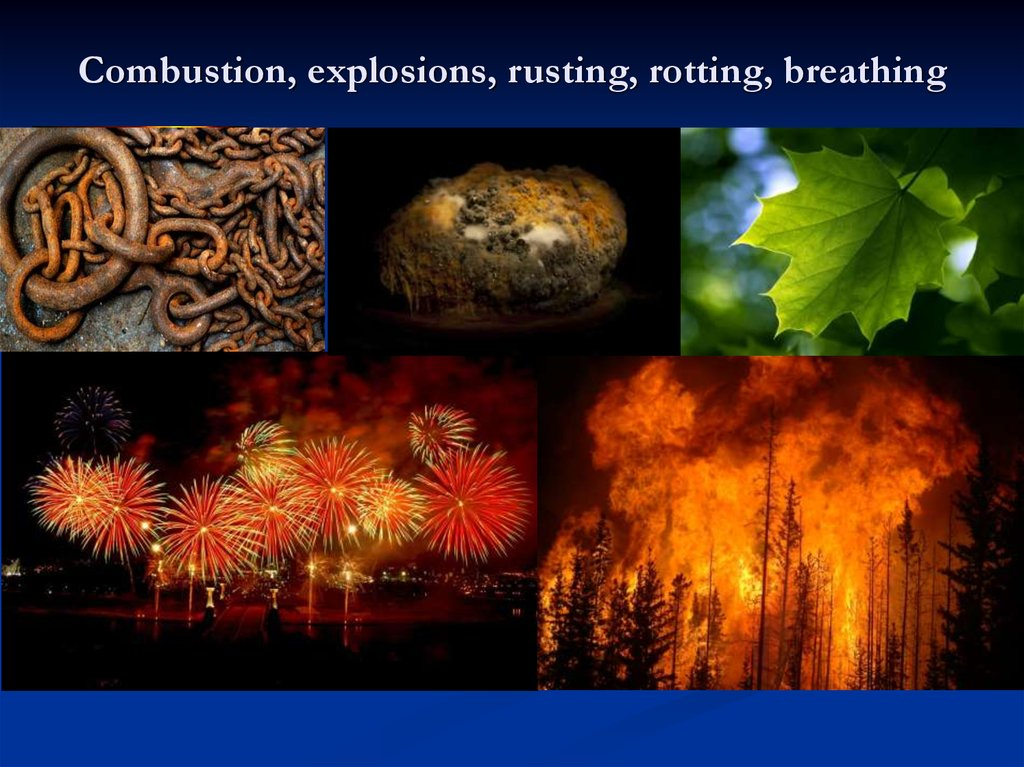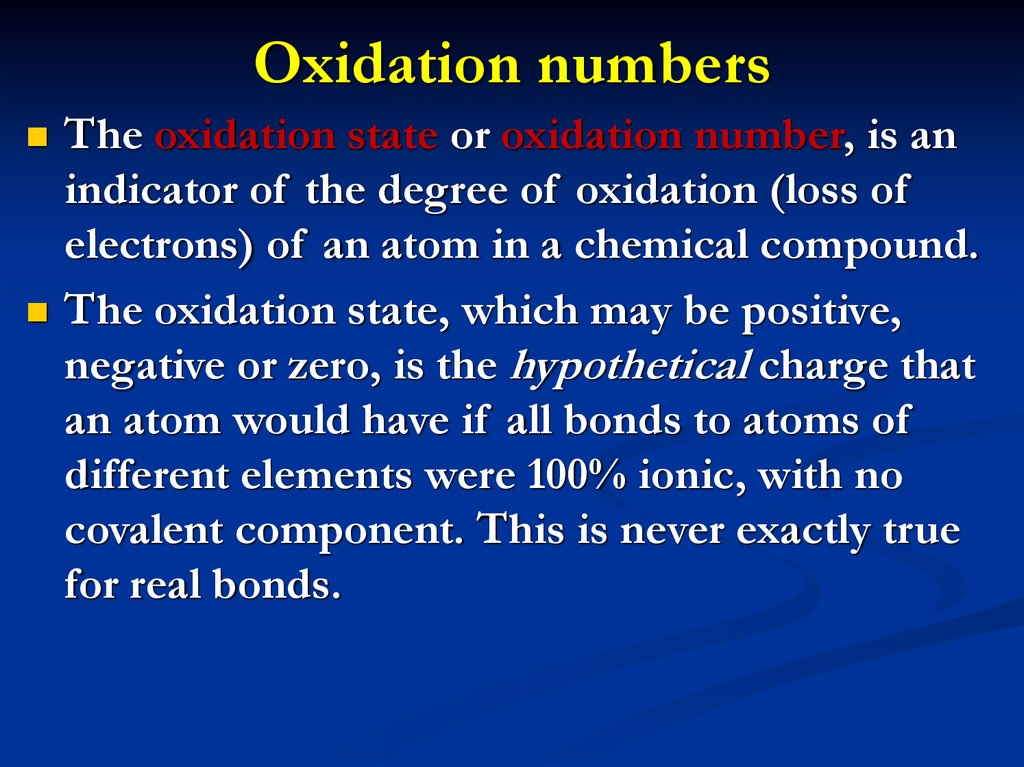# Redox Reactions

## 3. Oxidation numbers

The oxidation state or oxidation number, is an
indicator of the degree of oxidation (loss of
electrons) of an atom in a chemical compound.
The oxidation state, which may be positive,
negative or zero, is the hypothetical charge that
an atom would have if all bonds to atoms of
different elements were 100% ionic, with no
covalent component. This is never exactly true
for real bonds.

Fe(OH)2
Fe(OH)3
iron is in
oxidation state
+2
iron is in
oxidation state
+3

## 6. Determining the oxidation state or number

Any pure element—even if it forms diatomic molecules like
chlorine (Cl2)—has an oxidation state of zero.
For monatomic ions, the oxidation state is the same as the
charge of the ion. E.g., the chloride anion (Cl−) has an
oxidation state of −1, whereas the lithium cation (Li+) has an
oxidation state of +1.
The sum of oxidation states for all atoms in a polyatomic ion is
equal to the charge of the ion. Thus, the oxidation state of one
element can be calculated from the oxidation states of the other
elements.
The sum of the oxidation states of all atoms in a neutral
molecule must be zero.

## 7. Rules based on electronegativity

Fluorine in compounds has an oxidation state of −1.
Halogens other than fluorine have an oxidation state of −1
except when they are bonded to oxygen, to nitrogen, or to
another halogen that is more electronegative.
Hydrogen has an oxidation state of +1 except when bonded
to more electropositive elements such as sodium, aluminium,
and boron.
In compounds, oxygen typically has an oxidation state of −2.
Alkali metals have an oxidation state of +1 in virtually all of
their compounds.
Alkaline earth metals have an oxidation state of +2 in
virtually all of their compounds.

## 8. Definitions:

Oxidation is the loss of electrons.
Reduction is the gain of electrons.
Any reaction involving the transfer of electrons is an
oxidation-reduction (or redox) reaction
Oxidation cannot take place without reduction.
During a redox reaction, the oxidation numbers of
reactants will change.

## 9. Oxidizing agents

Substances that have the ability to oxidize other substances (cause
them to lose electrons) are known as oxidizing agents, oxidants,
or oxidizers. The oxidizing agent removes electrons from another
substance, and is thus itself reduced. And, because it "accepts"
electrons, the oxidizing agent is also called an electron acceptor.
Oxygen is the quintessential oxidizer.
Substances with elements in high oxidation states (e.g.,
concentrated sulfuric acid H2SO4, potassium permanganate
KMnO4, potassium dichromate(VI) K2Cr2O7, manganese(IV)
oxide MnO2)
or else highly electronegative elements (O2, F2, Cl2, Br2) that can
gain extra electrons by oxidizing another substance.

## 10. In the Thermit reaction, shown here, which substance is reduced and which is oxidized?

Aluminium (Al) removes the oxygen
atoms from the iron(III) oxide (Fe2O3).
The heat needed to start the reaction is
usually provided by a magnesium fuse.
2Al + Fe2O3 Al2O3 + 2Fe
The iron(III) ions are reduced and the
aluminium ions are oxidized.
This reaction produces much heat. It is
used in incendiary weapons and in
underwater welding.

## 11. Reducing agents

Substances that have the ability to reduce other substances
(cause them to gain electrons) are said to be reductive or
reducing and are known as reducing agents, reductants, or
reducers. They transfer electrons to another substance, and
are thus oxidized. And, because it "donates" electrons, the
reducing agent is also called an electron donor.
Electropositive elemental metals, such as Li, Na, Mg, Fe,
Zn, and Al. These metals donate or give away electrons
C, CO, H2

## 12. For any equation to be balanced:

1. The number of atoms of each type on the left side of
the arrow must equal the number of atoms of each
type to the right of the arrow.
2.
The total charges on the left side of the arrow must
equal the total charges to the right of the arrow.
3.
The electrons lost (during oxidation) must equal the
electrons gained (during reduction).

## 13. Types of redox reactions

1. Combination
General equation: A+B→AB
2 H2 + O2 = 2 H2O
2. Decomposition
General equation: AB → A + B
2Ag2O = 4Ag + O2 (heating)
(NH4)2Cr2O7 = N2 + Cr2O3 + 4H2O (heating)
Decomposition reactions are the reverse of combination reactions,
meaning they are the breakdown of a chemical compound into its
component elements.

## 14. Balancing the equations

K+Mn+7O4-2 + K+Cl- + H2SO4 = Cl20 + Mn+2SO4 + K2SO4 + H2O
Mn+7 + 5e- = Mn+2
reduction
2
2Cl- - 2e- = Cl20 oxidation
10
5
2 KMnO4 + 10KCl + 8H2SO4 = 5Cl2 + 2MnSO4 + 6K2SO4 + 8H2O

## 15. Types of redox reactions

Single Displacement
General equation: A + BC → AB + CA
Cl2 + 2 NaBr = 2 NaCl + Br2
2AgNO3 + Cu = Cu(NO3)2 + 2Ag

## 16. Types of redox reactions

Combustion
CH₄ + 2O₂ → CO₂+ 2H₂O + Q
Combustion reactions
always involve oxygen
and an organic fuel.

## 17. Types of redox reactions

Disproportionation
The same substances are both oxidized and reduced.
These are known as disproportionation reactions.
2 H2O2(aq) → 2 H2O(l) + O2(g)
In H2O2, oxygen has an oxidation state of -1.
In H2O, its oxidation state is -2,
and it has been reduced.
In O2 its oxidation state is 0,
and it has been oxidized.
Oxygen has been both oxidized and reduced in the reaction, making this
a disproportionation reaction.

## 18.

Chemistry IB – Chapter 10, pp.302-335
Balance the equations
NH3 + O2 = NO + H2O
CuO + NH3 = Cu + N2 + H2O
NO2 + H2O = HNO3 + NO
NH4NO3 = N2O + H2O
K2Cr2O7 + KI + H2SO4 = Cr2(SO4)3 + K2SO4 + I2 + H2O
KMnO4 + HCl = Cl2↑ + MnCl2 + KCl + H2O